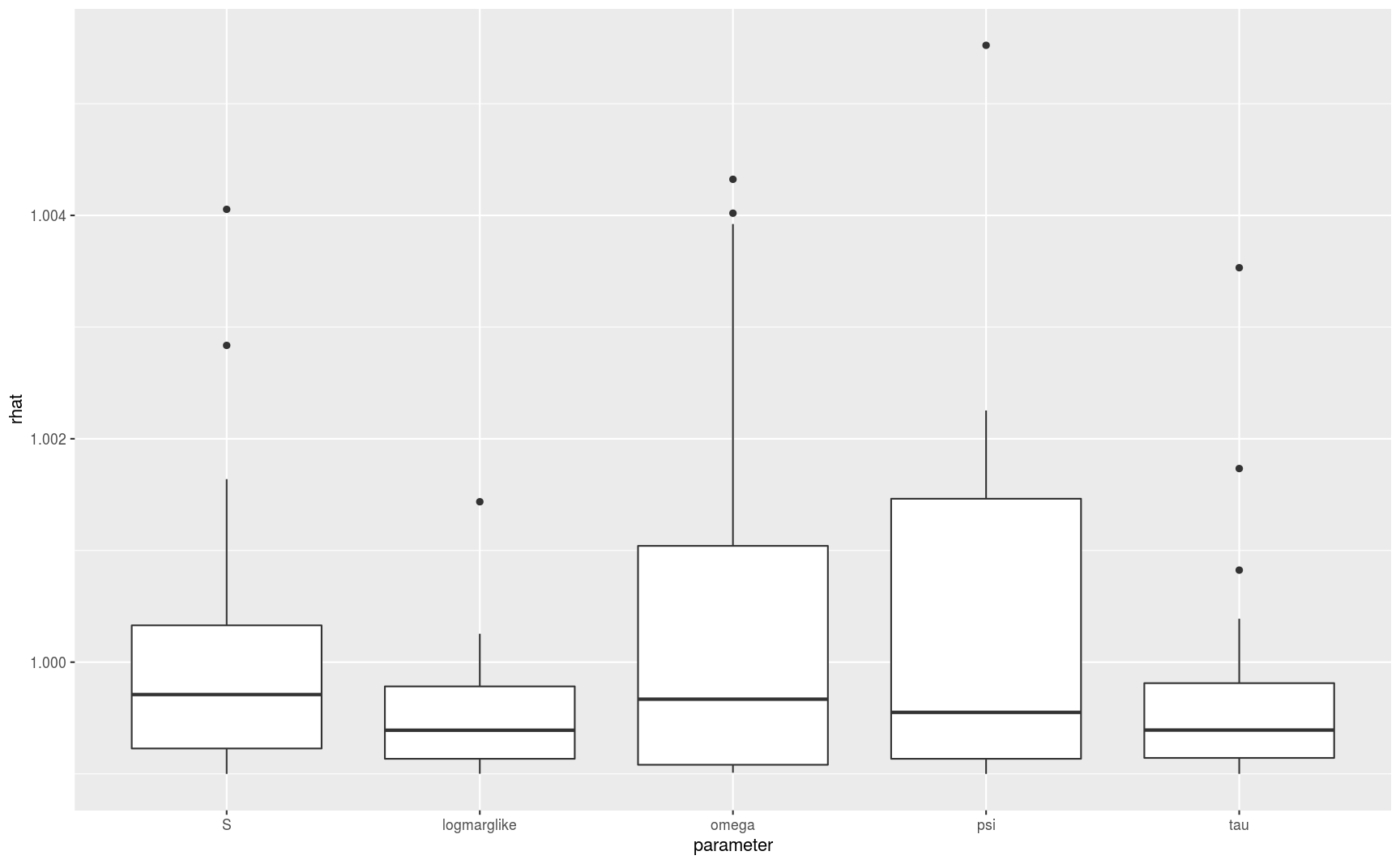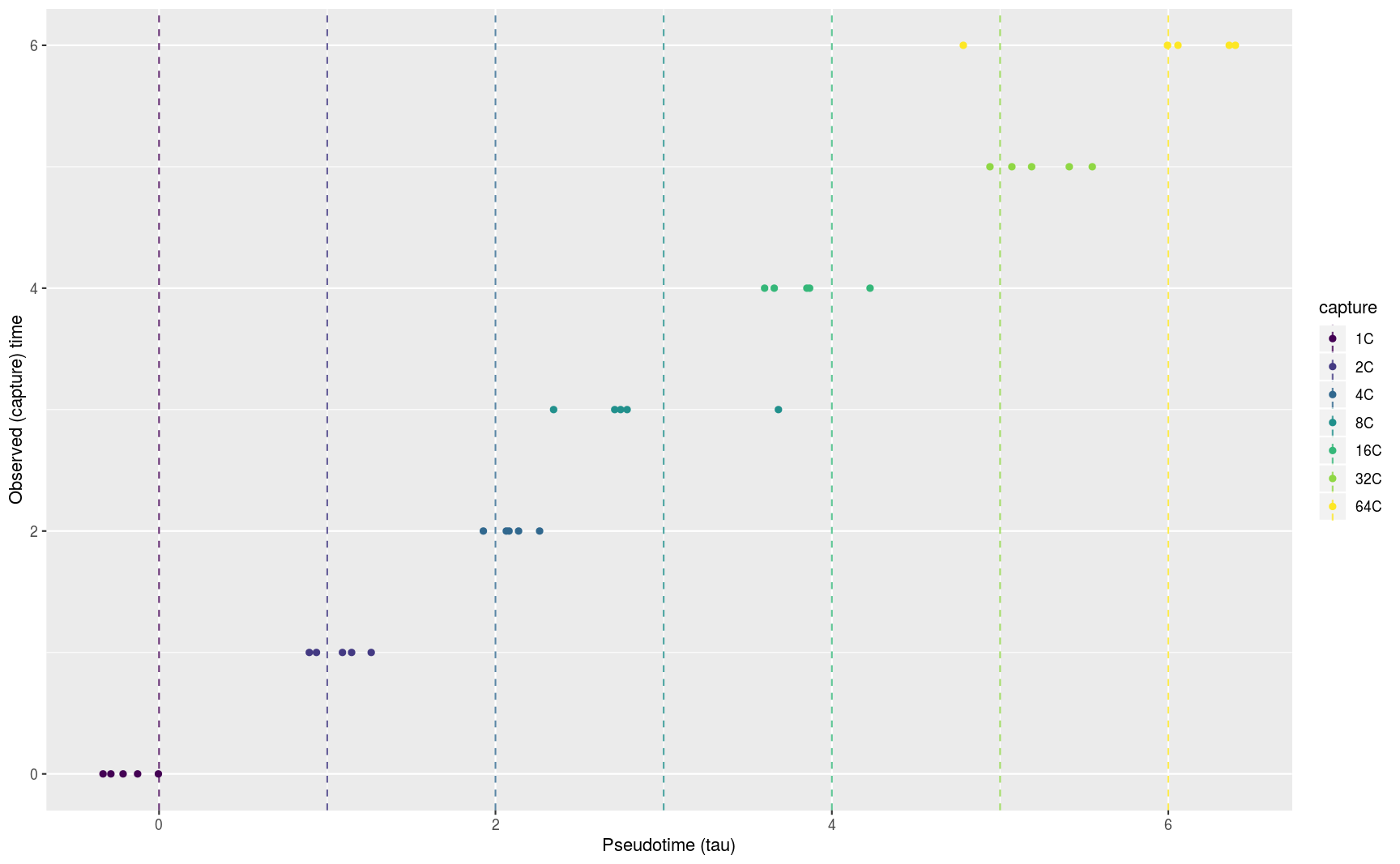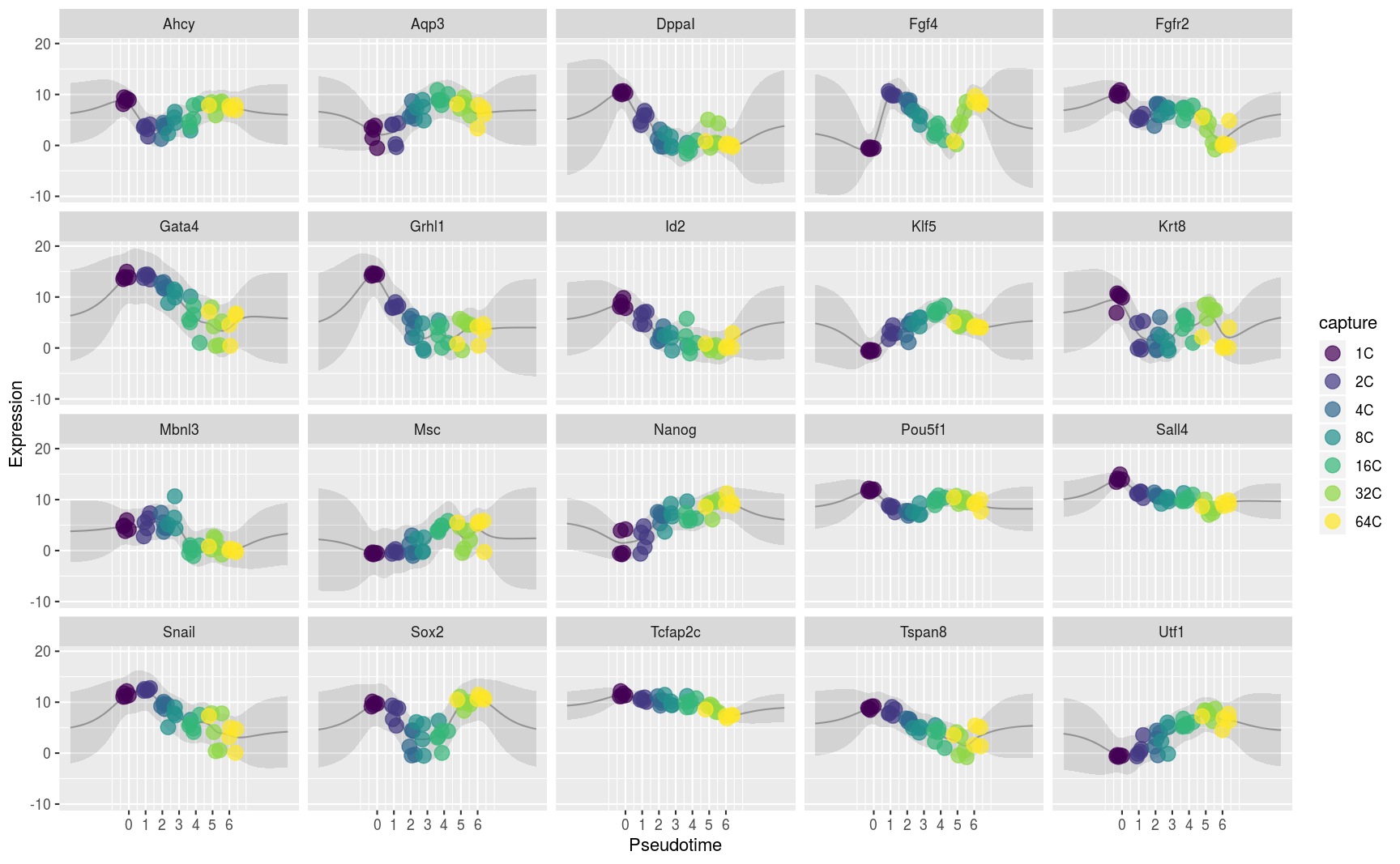# The DeLorean pseudotime estimation package

#### 2018-10-17

Our understanding of dynamical biological systems such as developmental processes or transitions into disease states is limited by our ability to reverse-engineer these systems using available data. Most medium- and high-throughput experimental protocols (e.g. single cell RNA-seq) are destructive in nature, generating cross-sectional time series in which it is not possible to track the progress of one cell through the system. In typical systems, individual cells progress at different rates and a sample’s experimental capture time will not accurately reflect how far it has progressed. In these cases, cross-sectional data can appear particularly noisy.

## Pseudotime

We propose a probabilistic model that uses smoothness assumptions to estimate and correct for this effect. Each cell is assigned a pseudotime that represents its progress through the system. These pseudotimes are related to but not determined by the cells’ experimental capture times. Replacing capture times with pseudotimes gives us a more representative view of the underlying system, improving downstream analyses.

## Model

We model the smoothness assumption on each gene’s expression profile $$y_g$$ using a Gaussian process over pseudotime. Gene-specific parameters in the covariance function represent intrinsic measurement noise $$\omega_g$$ and variation of the profile over time $$\psi_g$$. Each sample’s pseudotime $$\tau_c$$ is given a normal prior centred on its capture time. \begin{aligned} y_{g} &\sim \mathcal{GP}(\phi_g, \Sigma_g) \\ \Sigma_g(\tau_1, \tau_2) &= \psi_g \Sigma_\tau(\tau_1, \tau_2) + \omega_g \delta_{\tau_1,\tau_2} \\ \log \psi_g &\sim \mathcal{N}(\mu_\psi, \sigma_\psi) \\ \log \omega_g &\sim \mathcal{N}(\mu_\omega, \sigma_\omega) \\ \Sigma_\tau(\tau_1, \tau_2) &= \textrm{Matern}_{3/2}\bigg(r=\frac{|\tau_1 - \tau_2|}{l}\bigg) = (1 + \sqrt{3}r) \exp[-\sqrt{3}r] \\ \tau_c &\sim \mathcal{N}(k_c, \sigma_\tau) \end{aligned} This model is effectively a one-dimensional Gaussian process latent variable model with a structured prior on the latent variable (pseudotime).

## Example

Guo et al. assayed single cell expression values at 7 time points in mouse embryonic cells and the data is contained in the DeLorean package. We will load this data and analyse a subset of it corresponding to the epiblast lineage. First we must build a de.lorean object with the correct data. Load the data.

library(DeLorean)
library(dplyr)
data(GuoDeLorean)
# Limit number of cores to 2 for CRAN
options(DL.num.cores=min(default.num.cores(), 2))

Create a de.lorean object from the full data set.

dl <- de.lorean(guo.expr, guo.gene.meta, guo.cell.meta)

Estimate hyperparameters for the model from the whole data set. Here we set the width of the normal prior on the pseudotimes to be 0.5.

dl <- estimate.hyper(
dl,
sigma.tau=0.5,
length.scale=1.5,
model.name='exactsizes')

DeLorean also offers slight variations of the model, we could use model.name='lowrank' or model.name='exact'. See the documentation for estimate.hyper for more details.

### Choose cells

Choose a few cells from each capture point from the epiblast lineage.

num.at.each.stage <- 5
epi.sampled.cells <- guo.cell.meta %>%
filter(capture < "32C" |
"EPI" == cell.type |
"ICM" == cell.type) %>%
group_by(capture) %>%
do(sample_n(., num.at.each.stage))
dl <- filter_cells(dl, cells=epi.sampled.cells$cell) ### Choose genes We only have data for a few genes and can easily model them all which is typical for qPCR data. RNA-seq data often has far too many genes for the model to fit. In any case most are probably irrelevant. In these cases we recommend an analysis of variance across the capture times to choose those genes whose means vary most across time. These are most likely to be relevant for fitting the model. dl <- aov.dl(dl) The most temporally variable genes (by p-value) are at the head of the result: head(dl$aov)
#> # A tibble: 6 x 7
#>   gene    term       df sumsq meansq statistic  p.value
#>   <fct>   <chr>   <dbl> <dbl>  <dbl>     <dbl>    <dbl>
#> 1 DppaI   capture     6 494.    82.3      47.4 2.15e-13
#> 2 Tcfap2c capture     6  66.9   11.1      33.0 1.87e-11
#> 3 Grhl1   capture     6 648.   108.       27.1 1.99e-10
#> 4 Sall4   capture     6 130.    21.6      23.2 1.17e- 9
#> 5 Tspan8  capture     6 254.    42.3      21.8 2.37e- 9
#> 6 Sox2    capture     6 402.    66.9      20.5 4.66e- 9

The least temporally variable genes (by p-value) are at the tail of the result:

tail(dl$aov) #> # A tibble: 6 x 7 #> gene term df sumsq meansq statistic p.value #> <fct> <chr> <dbl> <dbl> <dbl> <dbl> <dbl> #> 1 Sox17 capture 6 39.9 6.64 2.23 0.0699 #> 2 Pdgfra capture 6 82.3 13.7 2.03 0.0948 #> 3 Atp12a capture 6 19.8 3.29 1.92 0.112 #> 4 Msx2 capture 6 52.9 8.82 1.88 0.119 #> 5 Eomes capture 6 49.8 8.30 1.80 0.135 #> 6 Tcfap2a capture 6 29.7 4.95 0.796 0.581 and for instance you could run the model on the 20 most variable genes by executing dl <- filter_genes(dl, genes=head(dl$aov, 20)\$gene)

otherwise do not call filter_genes and DeLorean will use all the genes.

### Fit the model

Now we have the data we can fit our model using Stan’s ADVI variational Bayes algorithm. To run the No-U-Turn sampler use method='sample'.

dl <- fit.dl(dl, method='vb')

### Examine convergence

If running a sampler, Stan provides $$\hat{R}$$ statistics that can aid detecting convergence problems. This makes no sense for ADVI but we show how to produce the boxplots here for users of the samplers.

dl <- examine.convergence(dl)
plot(dl, type='Rhat')### Estimated pseudotimes

Plot the pseudotimes from the best sample (best in the sense of highest likelihood). The prior means for the capture points are shown as dashed lines.

plot(dl, type='pseudotime')### Expression profiles

Plot the expression data over the pseudotimes from the best sample.

dl <- make.predictions(dl)
plot(dl, type='profiles')